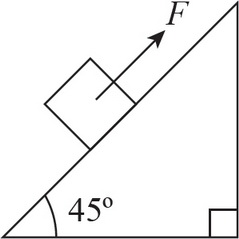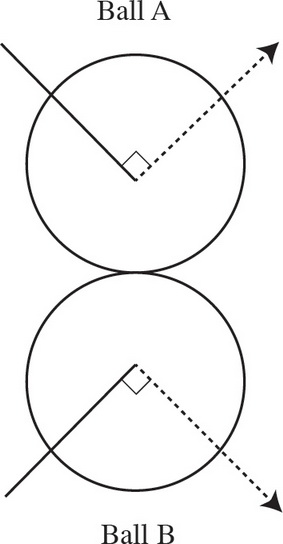# AP Physics 1 Multiple-Choice Practice Test 26

### Test Information10 questions18 minutes1. A box of mass m is sitting on an incline of 45° and it requires an applied force F up the incline to get the box to begin to move. What is the maximum coefficient of static friction?2. The graph above shows the velocities of two objects undergoing a head-on collision. Given that Object 2 has 4 times the mass of Object 1, which type of collision is it?3. The picture above depicts the collision of two balls of equal mass. Which arrow best indicates the direction of the impulse on Ball A from Ball B during the collision?

4. Which of the following best describes the relationship between the magnitude of the tension force, FT, in the string of a pendulum and the radial component of gravity that pulls antiparallel to the tension, Fg, radial? Assume that the pendulum is only displaced by a small amount.

5. Pretend someone actually managed to dig a hole straight through the center of the Earth all the way to the other side. If an object were dropped down that hole, which of the following would best describe its motion? Assume there is no drag or friction, the Earth is a perfect sphere, and the object cannot be destroyed.

6. A sound wave with frequency f travels through air at speed v. With what speed will a sound wave with frequency 4f travel through the air?

7. If an object's kinetic energy is doubled, what happens to its speed?

8. A toy car and a toy truck collide. If the toy truck's mass is double the toy car's mass, then, compared to the acceleration of the truck, the acceleration of the car during the collision will be

9. The Gravitron is a carnival ride that looks like a large cylinder. People stand inside the cylinder against the wall as it begins to spin. Eventually, it is rotating fast enough that the floor can be removed without anyone falling. Given that the coefficient of friction between a person's clothing and the wall is μ, the tangential speed is v, and the radius of the ride is r, what is greatest mass that a person can be to safely go on this ride?

10. In a spring-block oscillator, the maximum speed of the block is